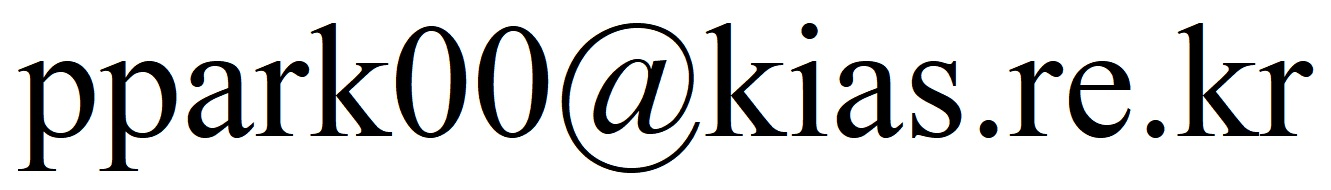## Jung-Tae Park (박정태)

### Research Fellow

Korea Institute for Advanced Study (KIAS)
85 Hoegiro, Dongdaemun-gu
Seoul 02455, Korea

Office: 1515
Email:### Education

Ph.D. in Mathematics, Seoul National University, September 2013 – February 2018   (Advisor: Sun-Sig Byun)
M.S. in Mathematics, Seoul National University, March 2007 – August 2009   (Advisor: Sun-Sig Byun)
B.S. in Mathematics, Pusan National University, March 2000 – February 2007

Curriculum Vitae   pdf

### Research Interests

Regularity theory for elliptic and parabolic differential equations

### Publications and Preprints

1. (with Pilsoo Shin) Regularity estimates for singular parabolic measure data problems with sharp growth, Submitted. Preprint
2. (with Sun-Sig Byun and Yumi Cho) Nonlinear gradient estimates for elliptic double obstacle problems with measure data, Submitted. Preprint
3. (with Karthik Adimurthi and Sun-Sig Byun) End point gradient estimates for quasilinear parabolic equations with variable exponent growth on nonsmooth domains, Calc. Var. Partial Differential Equations, to appear. Preprint
4. (with Sun-Sig Byun and Pilsoo Shin) Global regularity for degenerate/singular parabolic equations involving measure data, Calc. Var. Partial Differential Equations, 60(1), Paper No. 18, 32pp, 2021. Article, Preprint
5. (with Sun-Sig Byun) Optimal regularity for nonlinear elliptic equations with righthand side measure in variable exponent spaces, Indiana Univ. Math. J., 67(6), 2123–2150, 2018. Article, Preprint
6. (with Sun-Sig Byun) Global weighted Orlicz estimates for parabolic measure data problems: application to estimates in variable exponent spaces, J. Math. Anal. Appl., 467(2), 1194–1207, 2018. Article, Preprint
7. (with Karthik Adimurthi and Sun-Sig Byun) Sharp gradient estimates for quasilinear elliptic equations with $p(x)$ growth on nonsmooth domains, J. Funct. Anal., 274(12), 3411–3469, 2018. Article, Preprint
8. (with Sun-Sig Byun and Jihoon Ok) Regularity estimates for quasilinear elliptic equations with variable growth involving measure data, Ann. Inst. H. Poincaré Anal. Non Linéaire, 34(7), 1639–1667, 2017. Article, Preprint